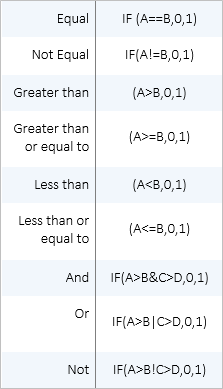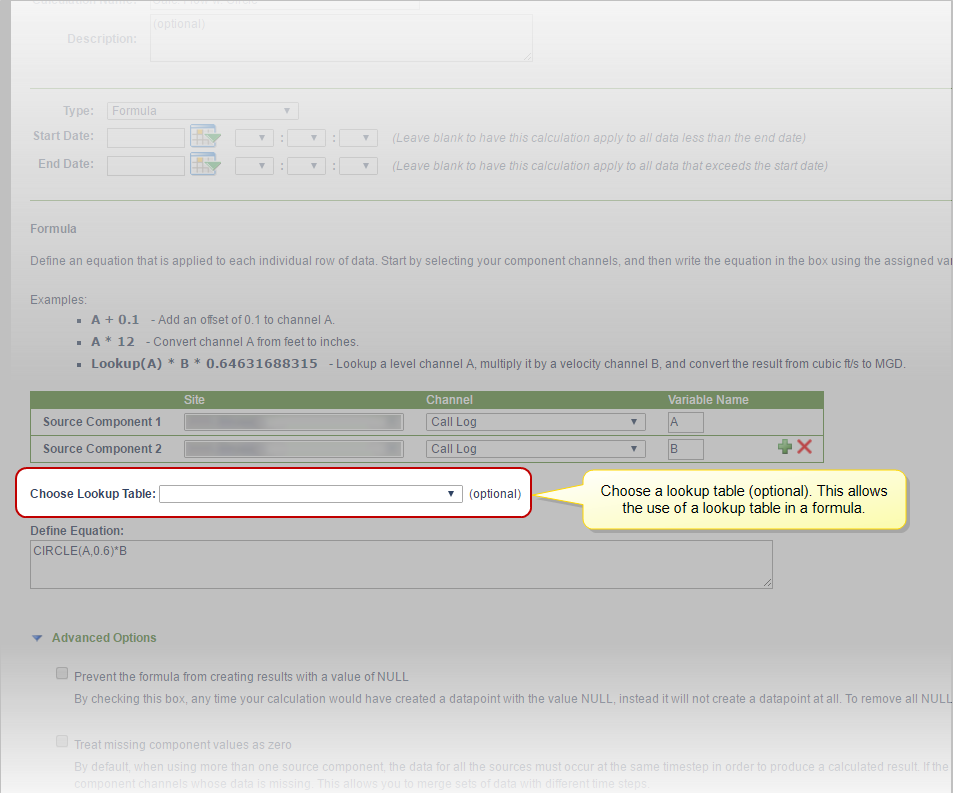The Formula feature in FACE allows you to define an equation using basic math,  trigonometric formulas, a Circle equation, and IF / ELSE statements; and apply the equation to each individual row of data from the source channel. All data derived from Formulas can be used in FlowWorks in the same ways as raw data channels - such as, calculated (virtual) data can be graphed and exported in a data table. This is achieved by way of first creating a Calculated Channel, and then appending a FACE formula.

Calculated Channels can also be configured with a predefined Function. FACE offers a host of commonly used functions for data analysis - choosing one of the many available functions allows for quick calculation setup, without having to continually go through the hassle of equation setup. To learn more about FACE functions, click here.

## Quick Reference Guide: Formula

The PDF file below provides a quick reference guide for FACE's basic capabilities using formula, as well as descriptions of the many available mathematical functions.

The circle function can be used to calculate flow when you know the diameter of a pipe and the depth of the material in that pipe. The calculation will return the area cross-section of the pipe occupied by the material. The syntax of this formula is: Circle(Depth, Diameter).

See article Formula Example: Computing Flow with the Circle Calculation.

## About Conditional Statements and Comparisons

Conditional statement (IF A>B THEN 0 ELSE 1) is written as: IF (A>B,0,1) where 0 and 1 may be a variable, constant, or equation. You may substitute any variable, constant or equation with the word NULL - for example: IF(A>B,0,null).

Valid comparisons include the following: